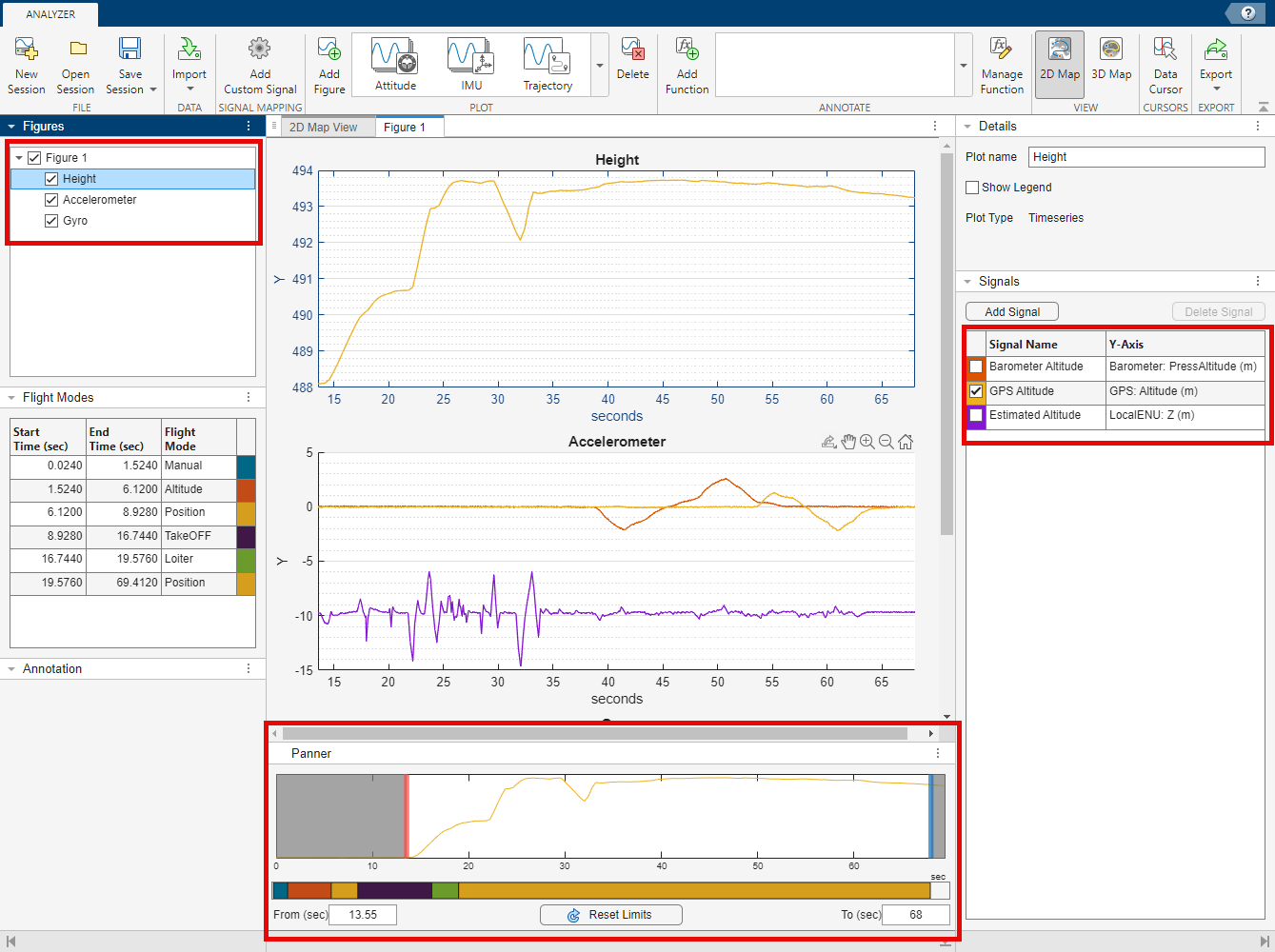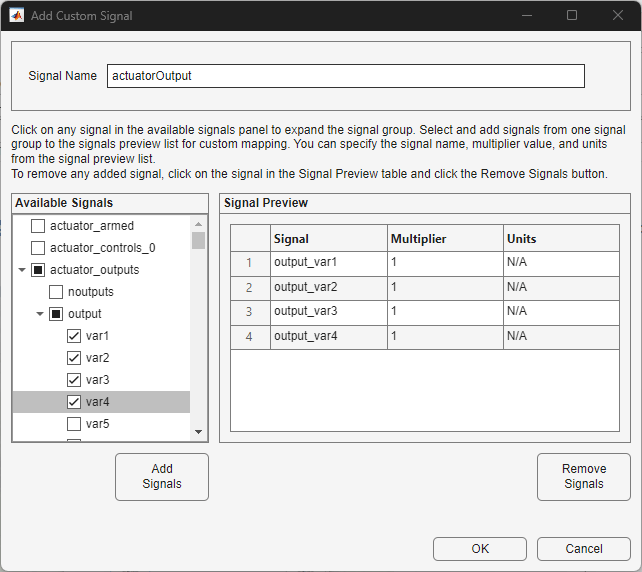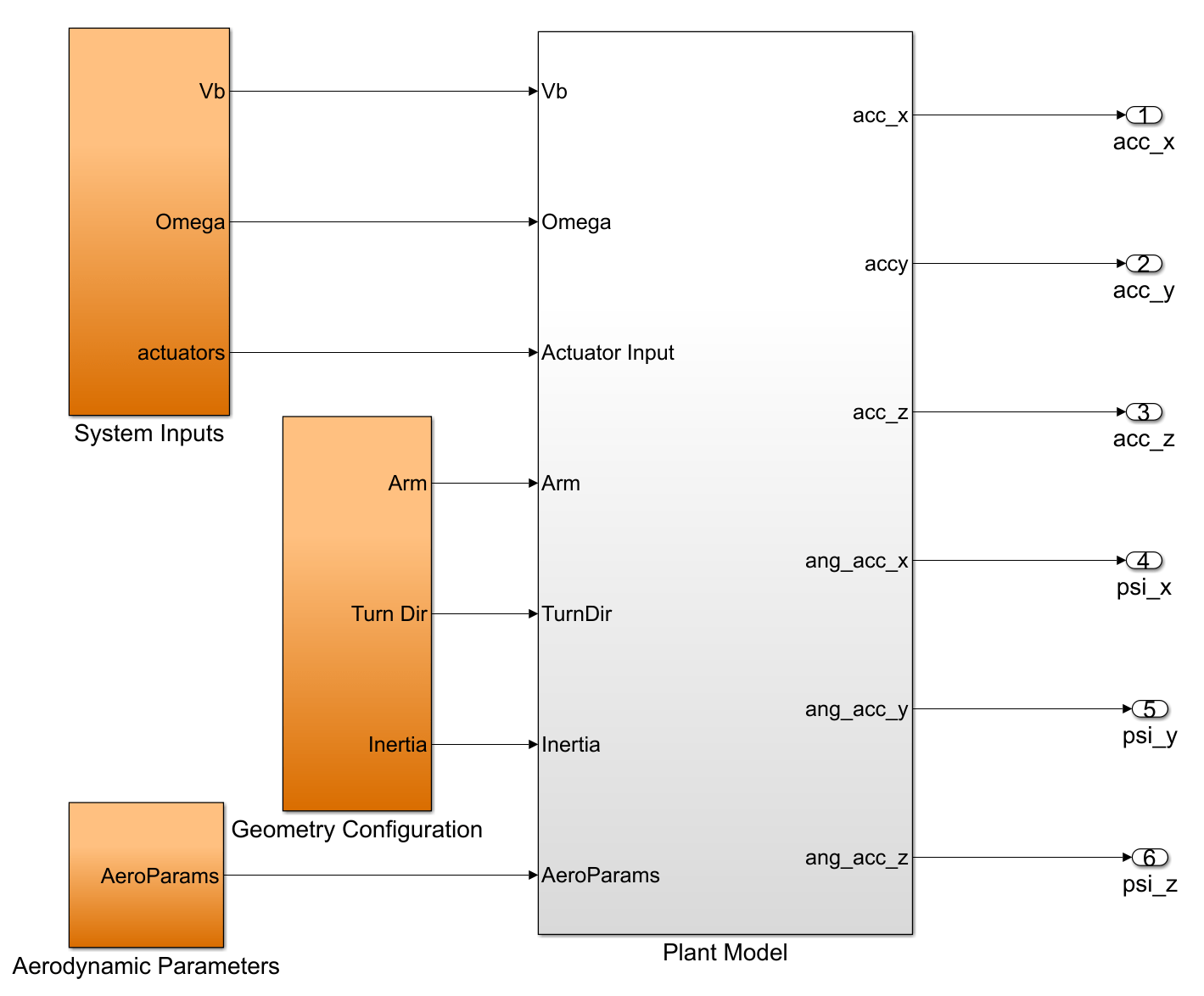# Aerodynamic Parameter Estimation Using Flight Log Data

This example shows how to estimate plant dynamics of your UAV from flight log data.

### Overview

When using simulated models in unmanned aerial vehicle (UAV) design, you must develop a digital-twin plant model that is as close as possible to the physical behavior of your UAV. A digital twin is a simulation-based representation of the UAV. During the design phase, guidance, navigation, and control (GNC) engineers can utilize this digital twin to design their control and guidance algorithms. Developing an accurate digital-twin model is an iterative process that requires flight tests and resolving any discrepancies between the simulation and physical data. Developing a simulation model can be an expensive process, which can require extensive wind-tunnel testing or fluid dynamics analysis. This example shows how to estimate the plant model dynamics from flight log data that contains measured sensor data. The process consists of these steps:

1. Process the flight log.

2. Set up the physics model.

3. Estimate physics parameters.

4. Obtain physics model fitted to flight data.### Visualize Flight Log and Identify Time of Interest

To visualize the flight log and identify a time of interest for parameter identification, use the `flightLogAnalyzer` function to open the Flight Log Analyzer app.

```flightLogAnalyzer ```

On the app toolstrip, select Import and click From ULOG. Then, select `quadrotor_model.ulg` and click Open. Next, click Add Figure and, in the Plot section of the toolstrip, select the Height and IMU plots. In the figure pane, select the Height plot and, in the Signals pane, clear the `Barometer Altitude` and `Estimated Altitude` signals. Observe that significant activity originates at the 13.5 second mark in both the accelerometer and gyroscope readings. The `GPS Altitude` signal in the height plot indicates that the UAV has lifted off at the same time as this activity.Define the start and end times for signal analysis based on this information.

```tStart = 13.55; tEnd = 68;```

### Prepare Flight Log Data

For identifying the system, you must resample all flight log data to the same time steps. Select a sampling interval of `0.01` seconds. Next, call the helper function `fetchSignalDataULog`, which imports and resamples the data to the specified time range.

`ug = ulogreader("quadrotor_model.ulg"); % Copyright 2021 Manuel Yves Galliker and Autonomous Systems Lab ETH Zurich `

Resample the data to the new frequency to get timeseries data `acc_x_T`, `acc_y_T`, and `acc_z_T` for the x, y, and z readings of the accelerometer, respectively.

```deltaT = 0.01; tSample = tStart:deltaT:tEnd; % Format Accelerometer, Gyroscope (output) and Actuator and Velocity (input) readings [acc_x_T,acc_y_T,acc_z_T,ang_vel_T,actuatorOutput,velocityNED] = exampleHelperFetchSignalDataULog(ug,tSample);```

Get the vehicle altitude from the flight data using the `exampleHelperRawData` helper function. Then, use the `exampleHelperSlerpData` helper function to interpolate the flight log orientation data using spherical interpolation to resample quaternion data at the new time steps.

```vehicleAttitude = exampleHelperRawData(ug,"vehicle_attitude","q",1:4); quatData = exampleHelperSlerpData(vehicleAttitude.Data,vehicleAttitude.Time,tSample);```

To compute the resulting forces at each rotor, you must solve for the local airspeed in the body frame defined as:

${\mathit{V}}_{\mathrm{local}\text{\hspace{0.17em}}\mathrm{airspeed}}$=${\mathit{V}}_{\mathit{b}}+{\omega }_{\mathit{b}}×\mathit{R}$,

where:

• ${\mathit{V}}_{\mathit{b}}$ — Velocity of the body frame, derived from the velocity in the north-east-down (NED) reference frame,${\mathit{V}}_{\mathrm{NED}}$.

• ${\omega }_{\mathit{b}}$ — Angular velocity of the body frame.

• $\mathit{R}$ — 3D rotor position.

To do this, convert the groundspeed in the flight log to the body frame using orientation data for each time sample. Note that this conversion assumes wind velocity is zero, but you can add a wind correction if a wind estimate is available.

```VNED = zeros(length(tSample),3); Vb = zeros(length(tSample),3); for ti = 1:1:length(tSample) % Get velocity in NED frame at current time step VNED(ti,1:3) = velocityNED.Data(ti,1:3); % Calculate body velocity using orientation data and velocity in NED frame q=quaternion([quatData(ti,1) quatData(ti,2) quatData(ti,3) quatData(ti,4)]); Vb(ti,1:3)=rotateframe(q,VNED(ti,1:3)); end```

Convert the body velocity into the timeseries format.

`Vb_T = timeseries(Vb,tSample);`

Differentiate the angular x, y, and z velocity data from the gyroscope to get the angular acceleration measurements. Convert all the angular acceleration data into `timeseries` objects.

```ang_acc_x_T = timeseries(gradient(ang_vel_T.Data(:,1))./deltaT,tSample); ang_acc_y_T = timeseries(gradient(ang_vel_T.Data(:,2))./deltaT,tSample); ang_acc_z_T = timeseries(gradient(ang_vel_T.Data(:,3))./deltaT,tSample);```

Set the actuator limits to the values actuator limits to a minimum value of `1000` and maximum value of `2000`, to normalize the actuator inputs between `0` and `1`.

```n_Actuator_Inputs = normalizeActuators(actuatorOutput.Data,1000,2000); n_Actuator_T = timeseries(n_Actuator_Inputs,tSample);```

### Set Up Parameter Estimation Model

Open the data dictionary `aeroParameters`, and then open the plant model `UAVQuadDynamics`. The data dictionary contains aerodynamic parameters to use as inputs to the plant model.

```plantDataDictionary = Simulink.data.dictionary.open("aeroParameters.sldd"); simModel = "UAVQuadDynamics.slx"; open_system(simModel)```The plant model is set up in Simulink® as a dynamical model of quadrotor dynamics to get the predicted response of the system. The model takes airspeed, rotational velocity, and actuator inputs and outputs a predicted system response containing linear and angular accelerations.

Initialize the aerodynamic parameters with some estimates. See the Appendix section for more information about these aerodynamic parameters.

```CD = 0.06; CT1 = -0.05; CT0 = 2; CDFuselage = [0.025 0.025 0.025]; CM0 = 1.5; CM1 = -0.01; CDM0 = 0.15; CDM1 = -0.04; CRM = 0.01;```

Open the `Aerodynamic Parameters` subsystem. The initialized aerodynamic parameters are inputs to the model in this subsystem.### Estimate Plant Dynamics

Tune the aerodynamic parameters to match the predicted response of the Simulink model to the measured system response. You can use Simulink Design Optimization™ to automate this process. First, obtain parameter estimates using the Parameter Estimator app.

#### Create Parameter Estimation Script

Open the dynamics plant model `UAVQuadDynamics` with the Parameter Estimator app.

```spetool("UAVQuadDynamics") ```

You can obtain the estimation setup by following the steps in this section, but if you want to skip to parameter estimation and tuning open the provided MAT file `flightdata_spesession` in the app and continue from the Tune Parameters section of this example.

From the app toolstrip, select New Experiment to open the Edit Experiment dialog box.

The first six fields in this box correspond to the six outputs of the dynamics plant model. Remove the `Constant2` field by clicking the X icon for that row. Then, specify the corresponding time-series output signals for each of the remaining six fields. For example, specify the `UAV/QuadDynamics/Plant_model:1 (acc_x)` field as `acc_x_T`, which is the linear acceleration in the x-direction. Note that, after specifying a field, the value displays as `<1x1 Signal, 5446 points>`. These are the correspondences between the fields and the dynamics plant model outputs.

• `acc_x` field — `acc_x_T` output

• `acc_y` field — `acc_y_T` output

• `acc_z` field — `acc_z_T` output

• `psi_x` field — `ang_acc_x_T` output

• `psi_y` field — `ang_acc_y_T` output

• `psi_z` field — `ang_acc_z_T` outputClick Select Parameters and select these parameters:

• CD

• CD Fuselage

• CDM0

• CDM1

• CM0

• CM1

• CRM

• CT0

• CT1Click OK to save your changes and close the Select mode variables dialog box. Click OK in the Edit Experiment dialog box.

Next, from the Parameter Estimator app toolstrip, click Estimate and select Generate MATLAB Function. The app generates a script to tune the parameters to the best values to fit to the flight log data.

#### Tune Parameters

Run the provided `parameterEstimationUAVQuadDynamics` script, which has been created using the same process as in the Create Parameter Estimation Script section, to automatically tune the parameters. Then update the parameters in the workspace.

```% Tune the parameters [pOpt,Info] = parameterEstimationQuadrotorDynamics; % Update the parameters in the workspace using the tuned parameters in pOpt for i=1:1:length(pOpt) eval(strcat(pOpt(i).Name,"=","[",num2str(pOpt(i).Value),"]")); end ```

Note that the process of tuning the parameters can take some time. You can instead load the tuned parameters from the `tunedParams` MAT file.

`load tunedParams.mat`

### Evaluate Fit to Log Data

Simulate the plant model which now uses the tuned parameters.

`simOut = sim("UAVQuadDynamics.slx")`
```### Starting serial model reference simulation build. ### Successfully updated the model reference simulation target for: RotorDragMoment ### Successfully updated the model reference simulation target for: ThrustForceModel Build Summary Simulation targets built: Model Action Rebuild Reason ============================================================================================= RotorDragMoment Code generated and compiled. RotorDragMoment_msf.mexa64 does not exist. ThrustForceModel Code generated and compiled. ThrustForceModel_msf.mexa64 does not exist. 2 of 2 models built (0 models already up to date) Build duration: 0h 0m 42.748s ```
```simOut = Simulink.SimulationOutput: logsout: [1x1 Simulink.SimulationData.Dataset] tout: [5446x1 double] yout: [1x1 Simulink.SimulationData.Dataset] SimulationMetadata: [1x1 Simulink.SimulationMetadata] ErrorMessage: [0x0 char] ```

Plot the x, y, and z acceleration output data from the model.

```% X Acceleration Plot figure set(gcf,color="w") hold on plot(simOut.tout,simOut.yout{1}.Values.Data(:),LineWidth=2) plot(acc_x_T) title("Accelerometer X Data") xlabel("Time (s)") ylabel("Measurement (m/s^2)") legend("Simulated","Flight Log") hold off``````% Y Acceleration Plot figure set(gcf,color="w") hold on plot(simOut.tout,simOut.yout{2}.Values.Data(:),LineWidth=2) plot(acc_y_T) title("Accelerometer Y Data") xlabel("Time (s)") ylabel("Measurement (m/s^2)") legend("Simulated","Flight Log") hold off``````% Z Acceleration Plot figure set(gcf,color="w") hold on plot(simOut.tout,simOut.yout{3}.Values.Data(:),LineWidth=2) plot(acc_z_T) title("Accelerometer Z Data") xlabel("Time (s)") ylabel("Measurement (m/s^2)") legend("Simulated","Flight Log") hold off```Calculate the root mean square error (RMSE) between the x-, y-, and z-data in the plant model and the x-, y-, and z-measurements in the flight log.

`RMSE_Acc_X = sqrt(mean((acc_x_T.Data-simOut.yout{1}.Values.Data(:)).^2))`
```RMSE_Acc_X = 0.0314 ```
`RMSE_Acc_Y = sqrt(mean((acc_y_T.Data-simOut.yout{2}.Values.Data(:)).^2))`
```RMSE_Acc_Y = 0.0431 ```
`RMSE_Acc_Z = sqrt(mean((acc_z_T.Data-simOut.yout{3}.Values.Data(:)).^2))`
```RMSE_Acc_Z = 0.1975 ```

The RMSE is low, which indicates that the tuned parameters fit the flight log well.

Discard the changes to the plant data dictionary and close the model.

```discardChanges(plantDataDictionary) close_system(simModel,0)```

### Summary

The estimated dynamics plant model fits the response of the given flight log data. As a next step, you can design a controller to control the obtained plant model dynamics. Also, because the current workflow uses readings obtained from derivatives of raw gyroscope data, you can better estimate angular acceleration dynamics by adding a filter to smooth the gyroscope data prior to identification. You can also utilize more flight logs to further refine your estimate of the plant dynamics.

### Appendix

This section contains a brief summary of the mathematical model used to estimate quadrotor dynamics. For a detailed study, see .

You can compute the predicted moment forces based on the state of the quadrotor at any timestep. These predictions depend on the aerodynamic parameters ${\mathit{C}}_{\mathrm{T0}}$, ${\mathit{C}}_{\mathrm{T1}}$, ${\mathit{C}}_{\mathit{D}}$, ${\mathit{C}}_{\mathrm{DM0}}$, ${\mathit{C}}_{\mathrm{DM1}}$, ${\mathit{C}}_{\mathrm{Dx}}$, ${\mathit{C}}_{\mathrm{Dy}}$, ${\mathit{C}}_{\mathrm{Dz}}$,${\mathit{C}}_{\mathrm{M0}}$, ${\mathit{C}}_{\mathrm{M1}}$, and ${\mathit{C}}_{\mathrm{RM}}\text{\hspace{0.17em}}$. The measured linear and angular acceleration are:

${\mathit{a}}_{\mathrm{meas}}=\left(\frac{{\mathit{F}}_{\mathit{b}}^{\mathrm{total}}}{\mathit{m}}\right)$, and

${\Psi }_{\mathrm{meas}}$=${\mathit{I}}^{-1}$(${\mathit{M}}_{\mathit{b}}^{\mathrm{total}}$-${\omega }_{\mathit{b}}$x$\mathit{I}$${\omega }_{\mathit{b}}$)

where:

${\mathit{a}}_{\mathrm{meas}}$ — Measured linear acceleration.

${\Psi }_{\mathrm{meas}}$ — Measured angular acceleration.

$\mathit{I}$— Moment of inertia of the UAV body.

${\omega }_{\mathit{b}}$ — Angular velocity in the body frame.

${\mathit{F}}_{\mathit{b}}^{\mathrm{total}}$ — Predicted force in the body frame.

${\mathit{M}}_{\mathit{b}}^{\mathrm{total}}$ — Predicted moment in the body frame.

${\omega }_{\mathit{b}}$ — Angular velocity in the body frame.

#### Force Computation

These are the contributions of forces. In this section, ${\mathit{v}}_{\mathit{l}}$ and ${\mathit{v}}_{\mathit{p}}$ are the parallel and perpendicular velocity components at each rotor, and ${\mathit{p}}_{\mathrm{rotor}}$ is the outward normal from the rotor.

The advance ratio at each rotor is:

`$\mathit{J}=\frac{{\mathit{v}}_{\mathit{l}}}{\eta \mathit{D}}$`

The thrust of each rotor is:

${\mathit{F}}_{\mathit{T}}^{\mathit{b}}$=$\rho {\eta }^{2}{\mathit{D}}^{4}$(${\mathit{C}}_{\mathrm{T0}}$+${\mathit{C}}_{\mathrm{T1}}\mathit{J}\right){\mathit{p}}_{\mathrm{rotor}}$

The rotor drag is:

${\mathit{F}}_{\mathit{D}}^{\mathit{b}}$=${-\mathit{C}}_{\mathit{D}}$$\eta$${\mathit{v}}_{\mathit{p}}$

The area of the fuselage is included in the drag coefficient. The fuselage drag in the x-, y-, and z-directions is:

${\mathit{F}}_{\mathrm{DFuselage}\text{\hspace{0.17em}}\mathit{x}|\mathit{y}|\mathit{z}}^{\mathit{b}}$|=-0.5*${\mathit{C}}_{\mathrm{Dx}|\mathit{y}|\mathit{z}}\rho {\mathit{V}}_{\mathit{x},\mathit{y},\mathit{z}}$$|{\mathit{V}}_{\mathit{x},\mathit{y},\mathit{z}}|$

#### Moment Computation

The model is also parameterized for moment contribution.

The moment contribution of thrust is:

${\mathit{M}}_{\mathit{T}}^{\mathit{b}}$=${\mathit{R}}_{\mathrm{arm}}\otimes \rho {\eta }^{2}{\mathit{D}}^{4}$(${\mathit{C}}_{\mathrm{M0}}$+${\mathit{C}}_{\mathrm{M1}}\mathit{J}\right){\mathit{p}}_{\mathrm{rotor}}$.

The rotor drag moment is:

${\mathit{M}}_{\mathit{D}}^{\mathit{b}}$=$\rho {\eta }^{2}{\mathit{D}}^{5}\left({\mathit{C}}_{\mathrm{Dm0}}+{\mathit{C}}_{\mathrm{Dm1}}\mathit{J}\right)$${\mathit{p}}_{\mathrm{rotor}}$.

The rotor rolling moment is:

${\mathit{M}}_{\mathit{R}}^{\mathit{b}}$=-${\mathit{C}}_{\mathrm{RM}}$$\eta$(TurnDir.${\mathit{p}}_{\mathrm{rotor}}$)${\mathit{v}}_{\mathit{p}}$.

You can obtain the linear acceleration measurement directly from accelerometer readings in the flight log, but there is no direct signal measuring angular acceleration in the flight log (${\Psi }_{\mathrm{meas}}$). Thus, you can use a time derivative of the gyroscope readings to obtain this measurement.

${\Psi }_{\mathrm{meas}}$=$\frac{\mathit{d}{\omega }_{\mathit{b}}}{\mathrm{dt}}$

Alternatively, if a separate measurement of angular acceleration is available, you can use that as a source for parameter estimation.

You can use the first two equations for ${\mathit{a}}_{\mathrm{meas}}$ and ${\Psi }_{\mathrm{meas}}$ to compute the predicted accelerations and angular momentum based on a parameterized Simulink model, and optimize the fit to the measured angular and linear acceleration.

### References

 Galliker, Manuel Yves. "Data-Driven Dynamics Modelling Using Flight Logs." June 24, 2021. https://doi.org/10.3929/ETHZ-B-000507495.